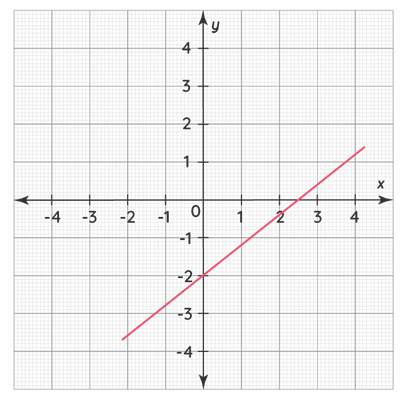Mathematics
Easy

Question

# John is trying to find the equation of the line(s) represented by the following graph.## 4x – 5y = 104x + 5y = 10Y = 4x + 2Y = 4x - 2Hint:

## The correct answer is: 4x – 5y = 10

### GIVEN-The given line passes through the points (0,-2) & (2.5,0) as shown in the given graph.TO FIND-Equation of the given line.SOLUTION-From the given graph, we observe that-(0,-2) & (2.5,0)We know that when a line passes through a given point, the coordinates of that point satisfies the equation of the said line.Hence, we substitute the coordiantes of both the point s in the given options to find the correct equation, for which LHS = RHS.a. 4x - 5y = 10We substitute (0,-2) in the given equation-           4x - 5y = 10∴ 4 (0) - 5 (-2) = 10∴          0 + 10 = 10∴                10 = 10∴             LHS = RHSWe substitute (2.5,0) in the given equation-             4x - 5y = 10∴ 4 (2.5) - 5 (0) = 10∴             10 - 0 = 10∴                  10 = 10∴               LHS = RHSSince both (0,-2) and (2.5,0) satisfy the given equation, 4x - 5y = 10 is the equation of the given line.Final Answer:-Option 'a' i.e. '4x - 5y = 10' is the correct answer to the given question.

Alternatively, we can use the 2 point formula to find the equation of the given line-
When we have 2 points that lie on a given line then we can find the equation of the said line by using the 2-point formula-
(y-y1) = (y2-y1) * (x-x1)
(x2-x1)
In the given question, x1 = 0, y1 = -2, x2 = 2.5 & y2 = 0
∴ (y-y1) = (y2-y1) * (x-x1)
(x2-x1)
∴ [y- (-2)] = [0 - (-2)] * (x - 0)
(2.5-0)
∴ (y + 2) =  2 *   x
2.5
∴ 2.5 * (y + 2) = 2x ................................... (Multiplying both sides by 2.5)
∴ 2.5y + 5 = 2x
∴ 5 = 2x - 2.5y
∴ 10 = 4x - 5y
i.e. 4x - 5y = 10

### Related Questions to study#### With Turito Foundation.#### Get an Expert Advice From Turito.